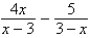# College Algebra

Mathematics

## Quiz 8 :PrerequisitesLooking for Calculus Homework Help?

## Quiz 8 :Prerequisites

Showing 1 - 20 of 24Determine which numbers in the set are irrational numbers.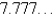,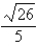, 0,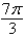,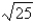,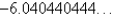,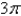Free
Multiple Choice

BApproximate the numbers and place the correct symbol (< or >) between them.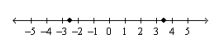Free
Multiple Choice

CUse inequality notation to describe the set. v is at least -16 but less than 10
Free
Multiple Choice

DEvaluate the expression.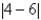Multiple ChoiceEvaluate the expression.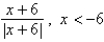Multiple ChoicePlace the correct symbol (<, >, or =) between the pair of real numbers.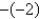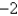Multiple ChoiceUse absolute value notation to describe the situation. The elevation at the bottom of a mountain is 417 feet above sea level. The elevation at the top of the mountain is 2187 feet above sea level. What is the height of the mountain?
Multiple ChoiceList the coefficients of the variable terms in the expression: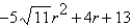Multiple ChoiceIdentify the rule of algebra illustrated by the statement.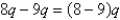Multiple ChoiceEvaluate the expression.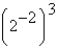Multiple ChoiceSimplify the expression.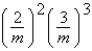Multiple ChoiceEvaluate the expression without using a calculator.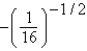Multiple ChoiceRationalize the denominator. Then simplify your answer.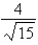Multiple ChoiceWrite the polynomial in standard form.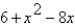Multiple ChoiceIdentify the degree and leading coefficient of the polynomial.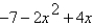Multiple ChoiceFactor the trinomial.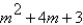Multiple ChoiceCompletely factor the expression.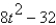Multiple ChoiceCompletely factor the expression.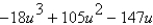Multiple ChoicePerform the division and simplify.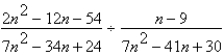Perform the subtraction and simplify.